# Matrix ring

full matrix ring

The ring of all square matrices of a fixed order over a ring $R$, with the operations of matrix addition and matrix multiplication. The ring of $(n \times n)$-dimensional matrices over $R$ is denoted by $R_n$ or $M_n(R)$. Throughout this article $R$ is an associative ring with identity.

The ring $R_n$ is isomorphic to the ring $\mathop{End}(M)$ of all endomorphisms of the free right $R$-module $M = R^n$, possessing a basis with $n$ elements. The identity matrix $E_n = \text{diag}(1,\ldots,1)$ is the identity in $R_n$. An associative ring $A$ with identity 1 is isomorphic to $R_n$ if and only if there is in $A$ a set of $n^2$ elements $e_{ij}$, $i,j=1,\ldots,n$, subject to the following conditions:

1) $e_{ij}e_{kl} = \delta_{jk} e_{il}$, $\sum_{i=1}^n e_{ii}e_{ii} = 1$;

2) the centralizer of the set of elements $e_{ij}$ in $A$ is isomorphic to $R$.

The centre of $R_n$ coincides with $Z(R) E_n$, where $Z(R)$ is the centre of $R$; for $n>1$ the ring $R_n$ is non-commutative.

The multiplicative group of the ring $R_n$ (the group of all invertible elements), called the general linear group, is denoted by $\mathop{GL}_n(R)$. A matrix from $R_n$ is invertible in $R_n$ if and only if its columns form a basis of the free right module of all $(n \times 1)$-dimensional matrices over $R$. If $R$ is commutative, then the determinant is defined as a multiplicative map from $R_n$ to $R$ and invertibility of a matrix $X$ in $R_n$ is equivalent to the invertibility of its determinant, $\det X$, in $R$. The isomorphism $R_{mn} \sim (R_m)_n$ holds.

The ring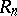is simple if and only ifis simple, for the two-sided ideals inare of the form, whereis a two-sided ideal in. An Artinian ring is simple if and only if it is isomorphic to a matrix ring over a skew-field (the Wedderburn–Artin theorem). If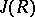denotes the Jacobson radical of the ring, then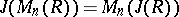. Consequently, every matrix ring over a semi-simple ringis semi-simple. Ifis regular (i.e. if for every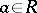there is a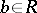such that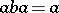), then so is. Ifis a ring with an invariant basis number, i.e. the number of elements in a basis of each free-module does not depend of the choice of the basis, thenalso has this property. The ringsandare equivalent in the sense of Morita (see Morita equivalence): The category of-modules is equivalent to the category of-modules. However, the fact that projective-modules are free does not necessarily entail that projective-modules are free too. For instance, ifis a field and, then there exist finitely-generated projective-modules which are not free.

How to Cite This Entry:
Matrix ring. Encyclopedia of Mathematics. URL: http://encyclopediaofmath.org/index.php?title=Matrix_ring&oldid=39107
This article was adapted from an original article by D.A. Suprunenko (originator), which appeared in Encyclopedia of Mathematics - ISBN 1402006098. See original article